# 2D Shapes on the Surface of 3D Shapes

2D Shapes on the Surface of 3D Shapes• 3D Shapes can be picked up and held in real life because they have length, width and depth.
• The flat surfaces of 3D shapes are called faces and are made up of 2D shapes.
• We can find the 2D shapes on the faces of 3D shapes by looking at the nets of these shapes.
• A cube has 6 square faces.
• A cylinder has 2 circular faces and a rectangular face that wraps around the outside.
• A cuboid has 6 rectangular faces.
• A cone has a circular face on the base.
• A triangular prism has 3 rectangular faces and 2 triangular faces.
• A square-based-pyramid has a square base and 4 triangular faces.
• A triangular-based pyramid has 4 triangular faces.

3D shapes have faces which are 2D Shapes.

We can rotate the shapes or look at their nets to find them.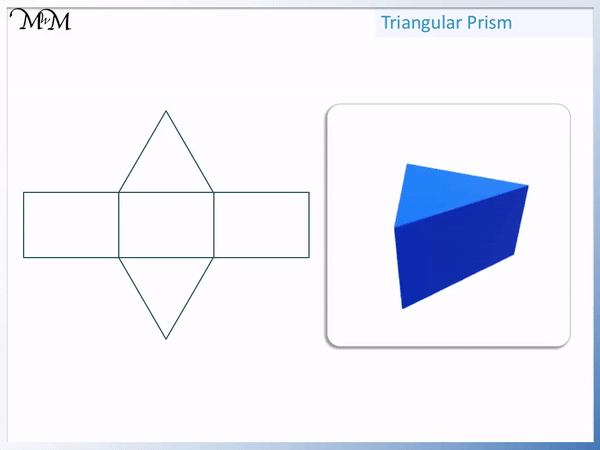• This is a triangular prism.
• It has 5 different faces in total.
• 3 faces are rectangles.
• The rectangles are on the left side, right side and the base.
• 2 faces are triangles.
• The triangles are on the front and back faces.#### Interactive 3D Shape Manipulatives

Cube   Cuboid   Cone   Triangular-Based Pyramid

# Identifying 2D Shapes on the Surface of 3D Shapes

## How to Identify 2D Shapes on 3D Shapes

‘2D’ is short for 2-dimensional. 2-dimensional shapes are flat. 2D shapes only exist on flat surfaces, such as screens or paper.

‘3D’ is short for 3-dimensional. 3-dimensional shapes can be picked up and exist in real life.

Many common 3D shapes are made up of flat surfaces called faces. These faces are often some of the 2D shapes that we know.

To identify 2D Shapes on the surface of 3D shapes, rotate the 3D shape to look at each face or use a net of the shape to help you.

We can see a list of some common 2D shapes on the surfaces of 3D shapes on the poster below:## Faces of a Cube

A cube is a 3D square. All of the edges on a cube are the same length and so, all of the faces are the same.A cube is made up of 6 square faces. These 6 square faces are identical and are all at right angles to each other. Each square face is directly opposite another square face.

We can see the 6 faces of a cube by rotating it as shown or we can look at its net shown below.This is one of the eleven different nets for a cube. Using this net, we can see that it is made up of 6 square faces.

## Faces of a Cylinder

A cylinder has 2 circular faces on the top and bottom. The side surface is a rectangle wrapped around the circles into a tubular shape.

A cylinder is a tube shaped 3d shape. It looks like a tin or can. It looks like a circle lifted upwards out of the paper it is drawn on.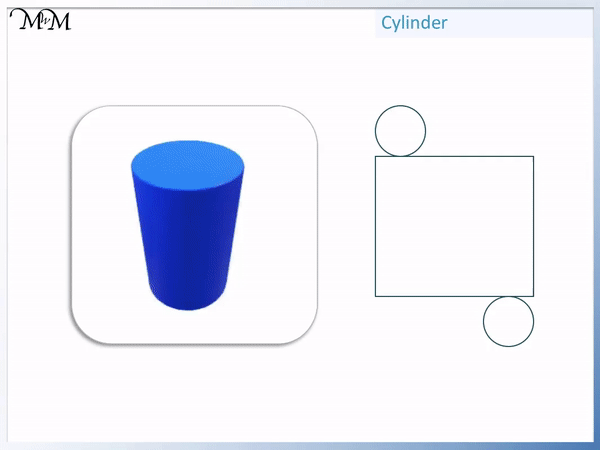We can see that the two circular faces are opposite to each other.

The side surface is curved, wrapped around the circular edges. Technically, faces must be flat and so this curved surface is not always counted as a face of a cylinder. We can see from the net below that this curved surface can be made from a rectangle wrapped around the side of the circles.

It is easiest to see the 2D shapes on the surfaces by looking at the net of the cylinder.

Here is the net of the cylinder with the faces shown.## Faces of a Cuboid

A cuboid has 4 rectangular faces. Each face is identical in size to the face opposite to it.

A cuboid is a box-shaped 3D shape. It is a 3D rectangle.A cuboid is a stretched cube. Therefore it has 6 faces just like a cube does.

We can see the 6 2D faces of a cuboid in the net below.## Faces of a Cone

A cone has one flat circular face as its base and one curved surface that wraps around the circular face.

Again, faces are technically flat and so the only face on a cone is the circle on its base. However, sometimes curved surfaces are included.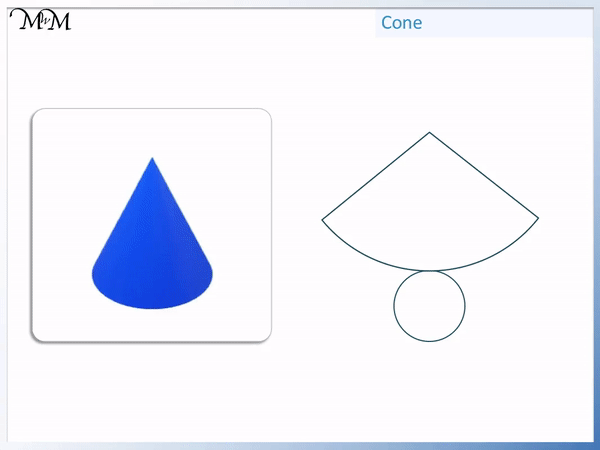We can see the net of the cone below, showing its circular 2D face.## Faces of a Triangular Prism

A triangular prism has two triangular faces surrounded by 3 rectangular faces.

The front face is a triangle. The back face is a triangle that is identical to the front face.We can see the net of a triangular prism below showing the 2D faces on its surface.

We have 3 rectangles and 2 triangles.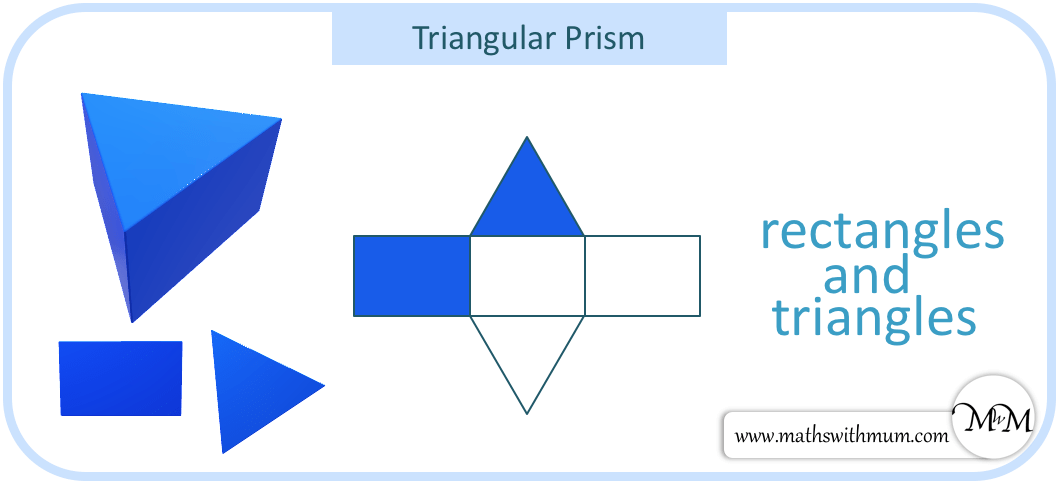## Faces of a Square-Based Pyramid

A square-based pyramid has one square face on its base and four triangular faces.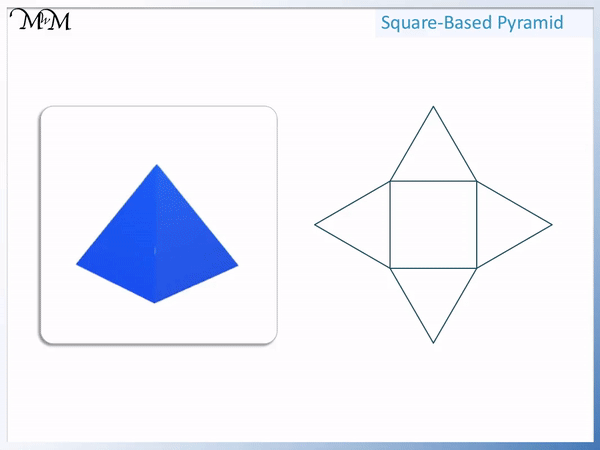A square has four sides and so the square base has a triangle attached to each edge. We can see the square surrounded by four triangles shown on the square-based pyramid net below.## Faces of a Triangular-Based Pyramid

A triangular-based pyramid has four triangular faces, one on its base and three on its sides.

We can see the 2D shapes on the triangular-based pyramid below.We can see the four 2D faces on the net of the triangular-based pyramid below.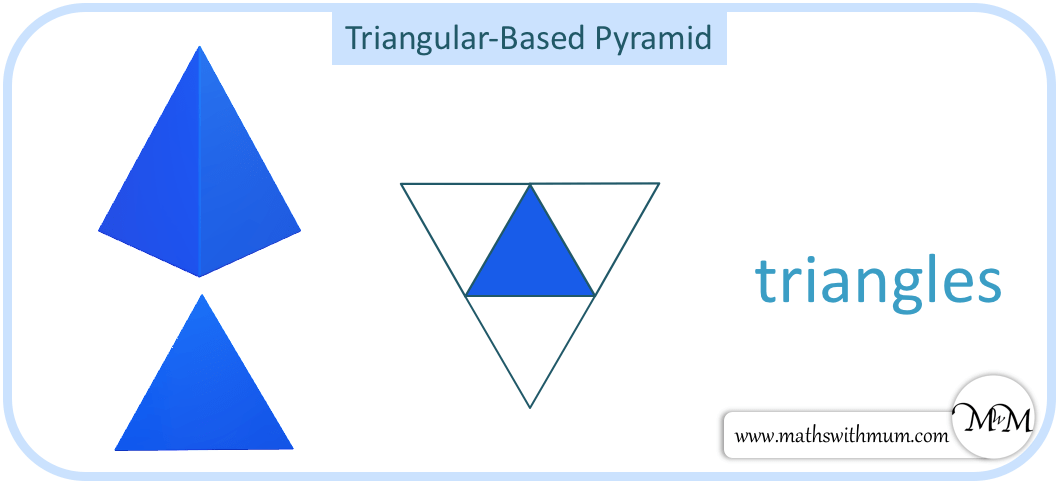Now try our lesson on Parallel Sides where we learn what parallel lines are and how to find them on shapes.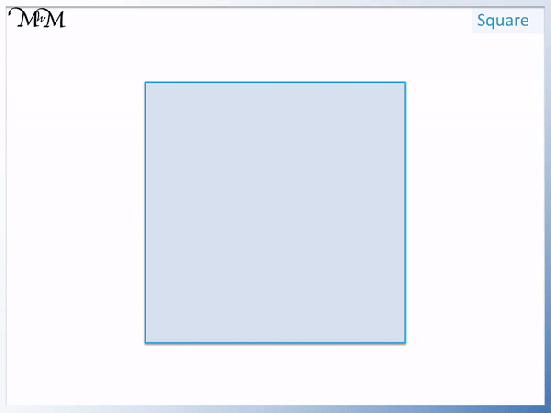error: Content is protected !!